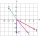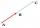# Vector

Calculate length of the vector v⃗ = (9.75, 6.75, -6.5, -3.75, 2).

Result

|v| =  14.18

#### Solution:Leave us a comment of this math problem and its solution (i.e. if it is still somewhat unclear...):Be the first to comment!#### Following knowledge from mathematics are needed to solve this word math problem:

Two vectors given by its magnitudes and by included angle can be added by our vector sum calculator. Do you want to convert length units? Pythagorean theorem is the base for the right triangle calculator.

## Next similar math problems:

1. SquarePoints A[-2,5] and B[-6,7] are adjacent vertices of the square ABCD. Calculate the area of the square ABCD.The rectangular crossroads comes passenger car and an ambulance, the ambulance left. Passenger car is at 43 km/h and ambulance 52 km/h. Calculate such a relative speed of the ambulance moves to the car.
3. TriangleTriangle KLM is given by plane coordinates of vertices: K[11, -10] L[10, 12] M[1, 3]. Calculate its area and itsinterior angles.
4. Three points 2The three points A(3, 8), B(6, 2) and C(10, 2). The point D is such that the line DA is perpendicular to AB and DC is parallel to AB. Calculate the coordinates of D.
5. Scalar productCalculate the scalar product of two vectors: (2.5) (-1, -4)An airplane leaves an airport and flies to west 120 miles and then 150 miles in the direction S 44.1°W. How far is the plane from the airport (round to the nearest mile)?
7. Find the 5Find the equation with center at (1,20) which touches the line 8x+5y-19=0
8. Two forcesTwo forces with magnitudes of 25 and 30 pounds act on an object at angles of 10° and 100° respectively. Find the direction and magnitude of the resultant force. Round to two decimal places in all intermediate steps and in your final answer.
9. Unit vector 2DDetermine coordinates of unit vector to vector AB if A[-6; 8], B[-18; 10].A ship travels 84 km on a bearing of 17°, and then travels on a bearing of 107° for 135 km. Find the distance of the end of the trip from the starting point, to the nearest kilometer.
11. Angle between vectorsFind the angle between the given vectors to the nearest tenth of a degree. u = (-22, 11) and v = (16, 20)
12. VectorsVector a has coordinates (8; 10) and vector b has coordinates (0; 17). If the vector c = b - a, what is the magnitude of the vector c?
13. Vector sumThe magnitude of the vector u is 12 and the magnitude of the vector v is 8. Angle between vectors is 61°. What is the magnitude of the vector u + v?
14. CuboidsTwo separate cuboids with different orientation in space. Determine the angle between them, knowing the direction cosine matrix for each separate cuboid. u1=(0.62955056, 0.094432584, 0.77119944) u2=(0.14484653, 0.9208101, 0.36211633)
15. Find the 10Find the value of t if 2tx+5y-6=0 and 5x-4y+8=0 are perpendicular, parallel, what angle does each of the lines make with the x-axis, find the angle between the lines?
16. LineLine p passing through A[-10, 6] and has direction vector v=(3, 2). Is point B[7, 30] on the line p?
17. Linear independenceDetermine if vectors u=(-4; -5) and v=(20; 25) are linear Linear dependent.